# XGBoost和LightGBM

1. 結構化資料：規整，維度固定；一般我們的表格資料都屬於結構化資料。
2. 非結構化資料：非規整，維度不固定；比如說一些文字、影象、音訊、視訊等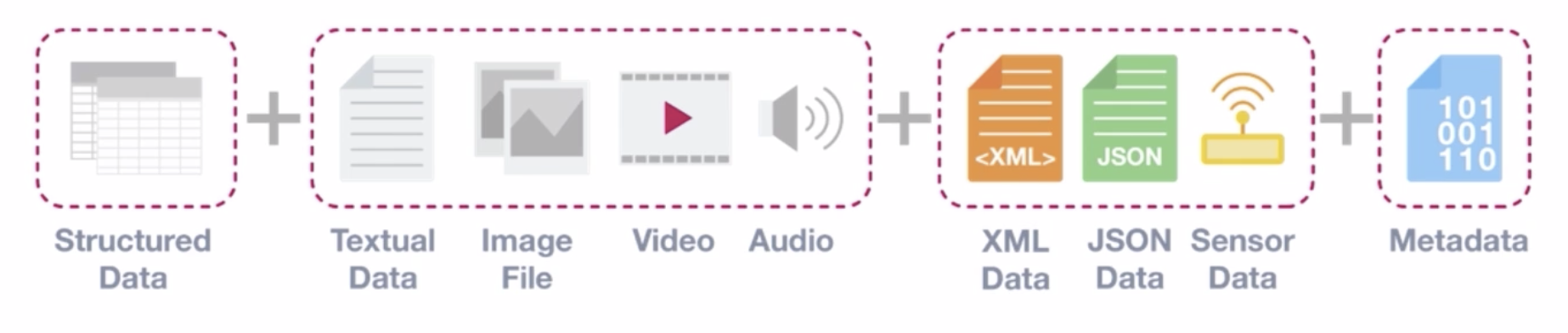1. 類別欄位較多
2. 聚合特徵較多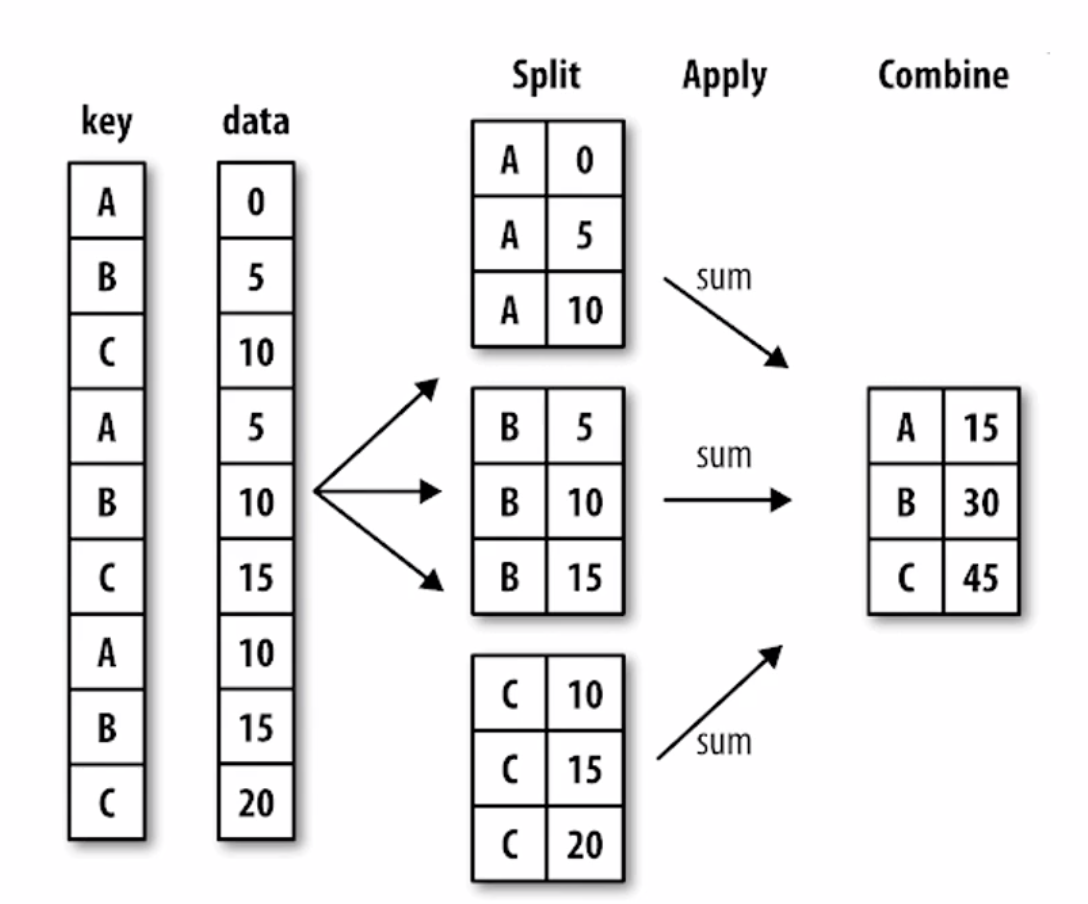XGBoost

XGBoost首先是樹模型，Xgboost就是由很多CART樹整合。一般有分類樹和迴歸樹，分類樹是使用資料集的特徵(維度)以及資訊熵或者基尼係數來進行節點分裂。對於迴歸樹則無法使用資訊熵和基尼係數來判定樹的節點分裂，包括預測誤差（常用的有均方誤差、對數誤差等）。而且節點不再是類別，是數值（預測值），那麼怎麼確定呢？有的是節點內樣本均值，有的是最優化算出來的比如XGBoost。

CART迴歸樹是假設樹為二叉樹，通過不斷將特徵進行分裂。比如當前樹結點是基於第j個特徵值進行分裂的，設該特徵值小於s的樣本劃分為左子樹，大於s的樣本劃分為右子樹。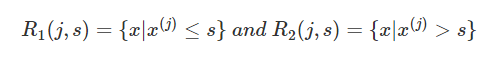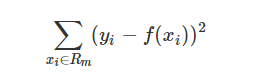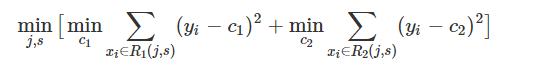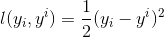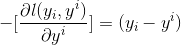XGBoostGBDT比較大的不同就是目標函式的定義XGBoost的目標函式如下圖所示(注意這裡是已經迭代切分之後的)：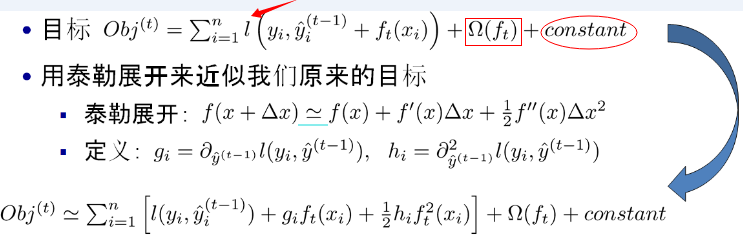• 紅色箭頭所指向的L 即為損失函式（比如平方損失函式：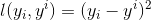，或邏輯迴歸損失函式：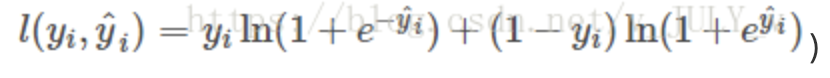• 紅色方框所框起來的是正則項（包括L1正則、L2正則）
• 紅色圓圈所圈起來的為常數項
• 對於，XGBoost利用泰勒展開三項，做一個近似。t是迭代次數

XGBoost的核心演算法思想

• 不斷地新增樹，不斷地進行特徵分裂來生長一棵樹，每次新增一個樹，其實是學習一個新函式，去擬合上次預測的殘差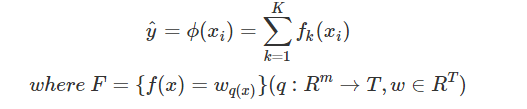• 當我們訓練完成得到k棵樹，我們要預測一個樣本的分數，其實就是根據這個樣本的特徵，在每棵樹中會落到對應的一個葉子節點，每個葉子節點就對應一個分數
• 最後只需要將每棵樹對應的分數加起來就是該樣本的預測值。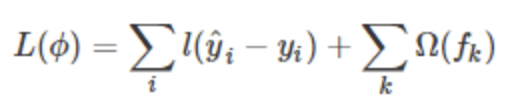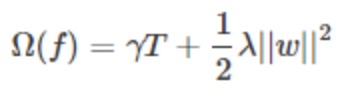T表示葉子節點的個數，w表示葉子節點的分數。直觀上看，目標要求預測誤差儘量小，且葉子節點T儘量少（γ控制葉子結點的個數），節點數值w儘量不極端（λ控制葉子節點的分數不會過大），防止過擬合。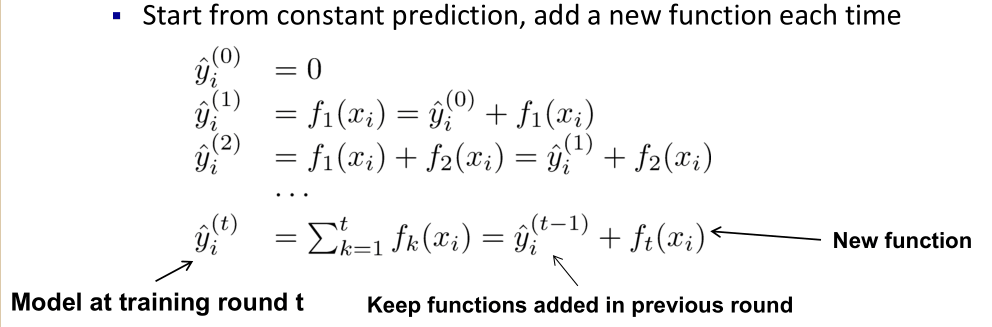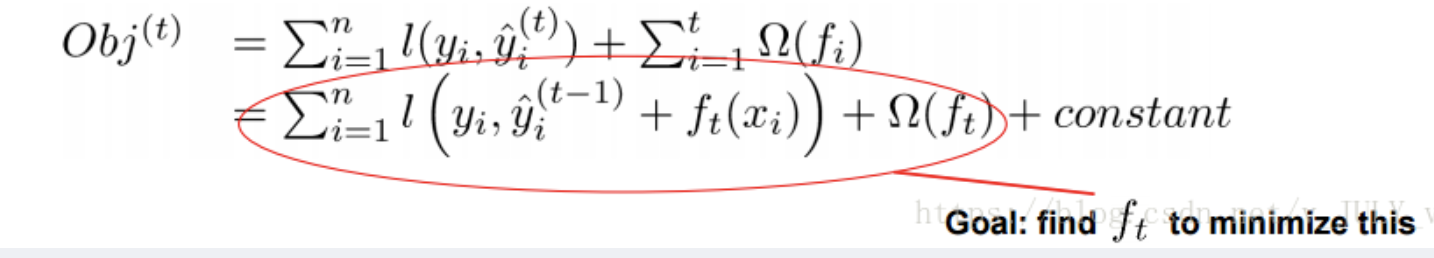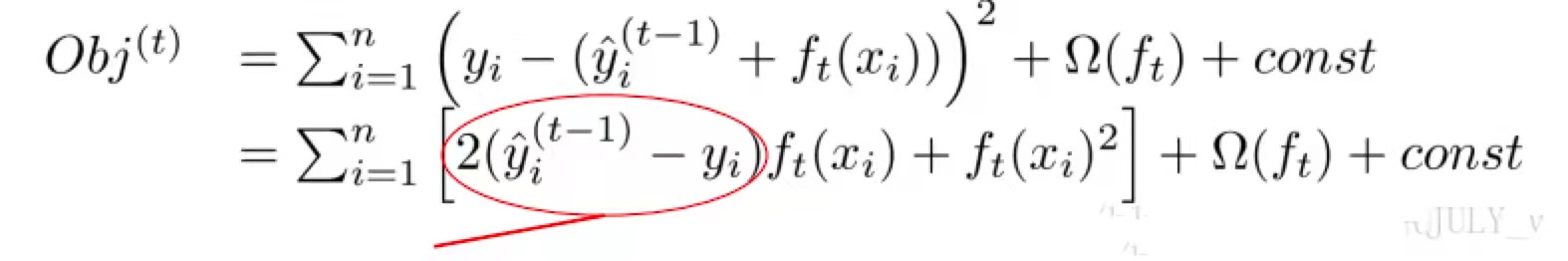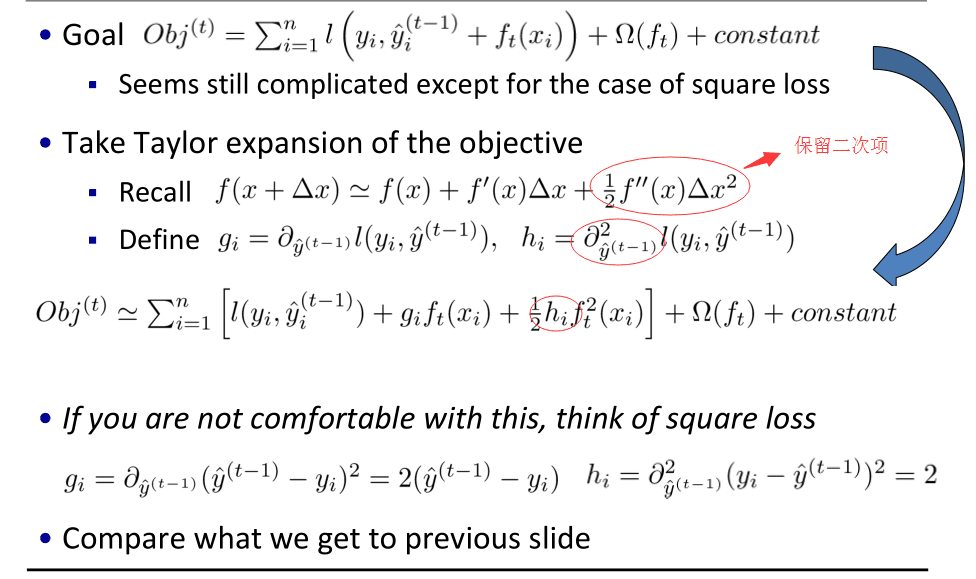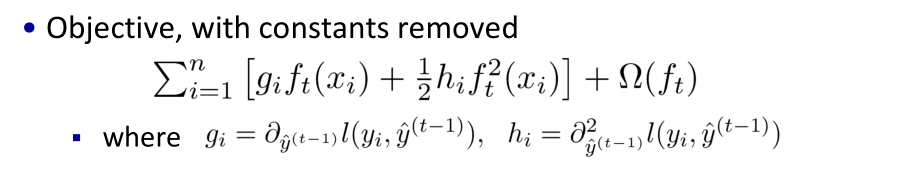XGBoost總的指導原則實質是把樣本分配到葉子結點會對應一個目標函式obj，優化過程就是目標函式obj優化。也就是分裂節點到葉子不同的組合，不同的組合對應不同目標函式obj，所有的優化圍繞這個思想展開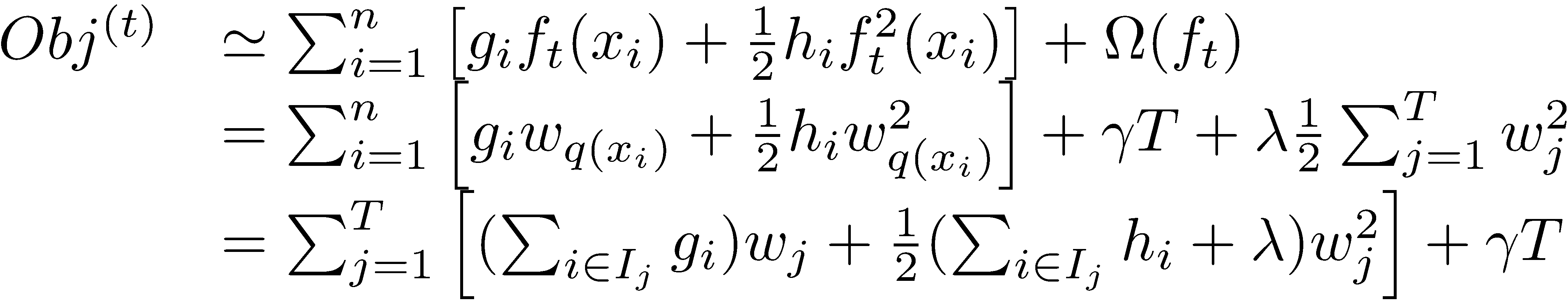• 一種是i - > n（樣本數）
• 一種是j -> T（葉子節點數）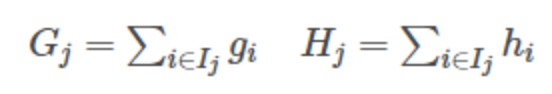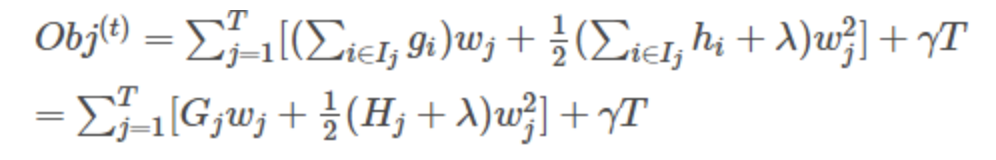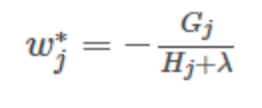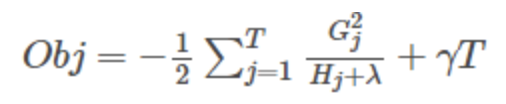```INPUT_TRAIN = "/Users/admin/Downloads/ga-customer-revenue-prediction/train.csv"
import os
import gc
import json
import time
from datetime import datetime
import timeit
import numpy as np
import pandas as pd
from pandas.io.json import json_normalize
import xgboost as xgb
from sklearn.model_selection import train_test_split
from sklearn.metrics import mean_squared_error
import warnings
warnings.filterwarnings('ignore')

if __name__ == "__main__":

pd.set_option('display.max_columns', 1000)
pd.set_option('display.width', 1000)
pd.set_option('display.max_colwidth', 1000)

# 匯入csv檔案
JSON_COLUMNS = ['device', 'geoNetwork', 'totals', 'trafficSource']
# 讀取檔案的資料，並將JSON_COLUMNS中的欄位讀取成json格式
dtype={'fullVisitorId': 'str'}, nrows=nrows)
# 將json格式的每一個欄位變成pandas表本身的欄位
for column in JSON_COLUMNS:
column_as_df = json_normalize(df[column])
column_as_df.columns = [f"{column}.{subcolumn}" for subcolumn in column_as_df.columns]
df = df.drop(column, axis=1).merge(column_as_df, right_index=True, left_index=True)
return df

def process_dfs(train_df, test_df):
# 資料預處理
print("Processing dfs...")
print("Dropping repeated columns...")
# 去重
columns = [col for col in train_df.columns if train_df[col].nunique() > 1]
train_df = train_df[columns]
test_df = test_df[columns]
trn_len = train_df.shape
merged_df = pd.concat([train_df, test_df])
merged_df['diff_visitId_time'] = merged_df['visitId'] - merged_df['visitStartTime']
merged_df['diff_visitId_time'] = (merged_df['diff_visitId_time'] != 0).astype('int')
del merged_df['visitId']
del merged_df['sessionId']
print("Generating date columns...")
format_str = "%Y%m%d"
merged_df['formated_date'] = merged_df['date'].apply(lambda x: datetime.strptime(str(x), format_str))
merged_df['WoY'] = merged_df['formated_date'].apply(lambda x: x.isocalendar())
merged_df['month'] = merged_df['formated_date'].apply(lambda x: x.month)
merged_df['quarter_month'] = merged_df['formated_date'].apply(lambda x: x.day // 8)
merged_df['weekday'] = merged_df['formated_date'].apply(lambda x: x.weekday())
del merged_df['date']
del merged_df['formated_date']
merged_df['formated_visitStartTime'] = merged_df['visitStartTime'].apply(
lambda x: time.strftime('%Y-%m-%d %H:%M:%S', time.localtime(x)))
merged_df['formated_visitStartTime'] = pd.to_datetime(merged_df['formated_visitStartTime'])
merged_df['visit_hour'] = merged_df['formated_visitStartTime'].apply(lambda x: x.hour)
del merged_df['visitStartTime']
del merged_df['formated_visitStartTime']
print("Encoding columns with pd.factorize()")
for col in merged_df.columns:
if col in ['fullVisitorId', 'month', 'quarter_month', 'weekday', 'visit_hour', 'Woy']:
continue
if merged_df[col].dtypes == object or merged_df[col].dtypes == bool:
merged_df[col], indexer = pd.factorize(merged_df[col])
print("Splitting back...")
train_df = merged_df[:trn_len]
test_df = merged_df[trn_len:]
return train_df, test_df

def preprocess():
target = train_df['totals.transactionRevenue'].fillna(0).astype('float')
target = target.apply(lambda x: np.log1p(x))
del train_df['totals.transactionRevenue']
train_df, test_df = process_dfs(train_df, test_df)
train_df.to_csv(TRAIN, index=False)
test_df.to_csv(TEST, index=False)
target.to_csv(Y, index=False)

preprocess()

def rmse(y_true, y_pred):
# 均方根誤差
return round(np.sqrt(mean_squared_error(y_true, y_pred)), 5)

# 匯入預處理完的資料
y_train = y_train[1:]
if drop_full_visitor_id:
X_train = X_train.drop('fullVisitorId', axis=1)
X_test = X_test.drop('fullVisitorId', axis=1)
return X_train, y_train, X_test

print(X.shape)
print(y.shape)
X_train, X_val, y_train, y_val = train_test_split(X, y, test_size=0.15, random_state=1)
print(f"Train shape: {X_train.shape}")
print(f"Validation shape: {X_val.shape}")
print(f"Test (submit) shape: {X_test.shape}")

def run_xgb(X_train, y_train, X_val, y_val, X_test):
params = {'objective': 'reg:linear',   # 線性迴歸的學習目標
'eval_metric': 'rmse',       # 均方根誤差校驗
'eta': 0.001,                # 更新過程中用到的收縮步長
'max_depth': 10,             # 樹最大深度
'subsample': 0.6,            # 用於訓練模型的子樣本佔整個樣本集合的比例
'colsample_bytree': 0.6,     # 在建立樹時對特徵取樣的比例
'alpha': 0.001,              # L1正則化項
'random_state': 42,          # 隨機種子
'silent': True}              # 列印模式
xgb_train_data = xgb.DMatrix(X_train, y_train)
xgb_val_data = xgb.DMatrix(X_val, y_val)
xgb_submit_data = xgb.DMatrix(X_test)
# num_boost_round進行2000次迭代，evals用於對訓練過程中進行評估列表中的元素
# early_stopping_rounds早期停止次數100，驗證集的誤差迭代到一定程度在100次內不能再繼續降低，就停止迭代。
# verbose_eval進行500次迭代輸出一次
model = xgb.train(params, xgb_train_data, num_boost_round=2000,
evals=[(xgb_train_data, 'train'), (xgb_val_data, 'valid')],
early_stopping_rounds=100, verbose_eval=500)
y_pred_train = model.predict(xgb_train_data, ntree_limit=model.best_ntree_limit)
y_pred_val = model.predict(xgb_val_data, ntree_limit=model.best_ntree_limit)
y_pred_submit = model.predict(xgb_submit_data, ntree_limit=model.best_ntree_limit)
print(f"XGB : RMSE val: {rmse(y_val, y_pred_val)}  - RMSE train: {rmse(y_train, y_pred_train)}")
return y_pred_submit, model

start_time = timeit.default_timer()
xgb_preds, xgb_model = run_xgb(X_train, y_train, X_val, y_val, X_test)
print('total time is:' + str(timeit.default_timer() - start_time))
```

``````Loading /Users/admin/Downloads/ga-customer-revenue-prediction/train.csv
Processing dfs...
Dropping repeated columns...
Generating date columns...
Encoding columns with pd.factorize()
Splitting back...
(903653, 31)
(903653,)
Train shape: (768105, 31)
Validation shape: (135548, 31)
Test (submit) shape: (804684, 31)
[06:20:31] WARNING: /Users/travis/build/dmlc/xgboost/src/objective/regression_obj.cu:171: reg:linear is now deprecated in favor of reg:squarederror.
[06:20:31] WARNING: /Users/travis/build/dmlc/xgboost/src/learner.cc:573:
Parameters: { "silent" } might not be used.

This may not be accurate due to some parameters are only used in language bindings but
passed down to XGBoost core.  Or some parameters are not used but slip through this
verification. Please open an issue if you find above cases.

	train-rmse:2.02025	valid-rmse:2.02937
	train-rmse:1.81049	valid-rmse:1.83542
	train-rmse:1.68934	valid-rmse:1.73293
	train-rmse:1.61610	valid-rmse:1.67851
	train-rmse:1.56843	valid-rmse:1.64969
XGB : RMSE val: 1.6497  - RMSE train: 1.5682
total time is:669.2252929020001``````

XGBoost引數

General Parameters

• booster [default=gbtree]

• silent [default=0]

• nthread [default to maximum number of threads available if not set]

XGBoost執行時的執行緒數。預設值是當前系統可以獲得的最大執行緒數

• num_pbuffer [set automatically by xgboost, no need to be set by user]

size of prediction buffer, normally set to number of training instances. The buffers are used to save the prediction results of last boosting step.

• num_feature [set automatically by xgboost, no need to be set by user]

boosting過程中用到的特徵維數，設定為特徵個數。XGBoost會自動設定，不需要手工設定。
Booster Parameters

• eta [default=0.3]

• gamma [default=0]

minimum loss reduction required to make a further partition on a leaf node of the tree. the larger, the more conservative the algorithm will be.
range: [0,∞]

• max_depth [default=6]

• min_child_weight [default=1]

• max_delta_step [default=0]

Maximum delta step we allow each tree’s weight estimation to be. If the value is set to 0, it means there is no constraint. If it is set to a positive value, it can help making the update step more conservative. Usually this parameter is not needed, but it might help in logistic regression when class is extremely imbalanced. Set it to value of 1-10 might help control the update

• subsample [default=1]

• colsample_bytree [default=1]

• objective [ default=reg:linear ]

1. “reg:linear” –線性迴歸。
2. “reg:logistic” –邏輯迴歸。
3. “binary:logistic”–二分類的邏輯迴歸問題，輸出為概率。
4. “binary:logitraw”–二分類的邏輯迴歸問題，輸出的結果為wTx。
5. “count:poisson”–計數問題的poisson迴歸，輸出結果為poisson分佈。在poisson迴歸中，max_delta_step的預設值為0.7。(used to safeguard optimization)
6. “multi:softmax” –讓XGBoost採用softmax目標函式處理多分類問題，同時需要設定引數num_class（類別個數）
7. “multi:softprob” –和softmax一樣，但是輸出的是ndata * nclass的向量，可以將該向量reshape成ndata行nclass列的矩陣。沒行資料表示樣本所屬於每個類別的概率。
8. “rank:pairwise”–set XGBoost to do ranking task by minimizing the pairwise loss
• base_score [ default=0.5 ]

the initial prediction score of all instances, global bias

• eval_metric [ default according to objective ]

The choices are listed below:

1. “rmse”: root mean square error
2. “logloss”: negative log-likelihood
3. “error”: Binary classification error rate. It is calculated as #(wrong cases)/#(all cases). For the predictions, the evaluation will regard the instances with prediction value larger than 0.5 as positive instances, and the others as negative instances.
4. “merror”: Multiclass classification error rate. It is calculated as #(wrong cases)/#(all cases).
5. “mlogloss”: Multiclass logloss
6. “auc”: Area under the curve for ranking evaluation.
7. “ndcg”:Normalized Discounted Cumulative Gain
8. “map”:Mean average precision
9. “ndcg@n”,”map@n”: n can be assigned as an integer to cut off the top positions in the lists for evaluation.
10. “ndcg-”,”map-”,”ndcg@n-”,”map@n-”: In XGBoost, NDCG and MAP will evaluate the score of a list without any positive samples as 1. By adding “-” in the evaluation metric XGBoost will evaluate these score as 0 to be consistent under some conditions. training repeatively
11. “gamma-deviance”: [residual deviance for gamma regression]
• seed[ default=0 ]

random number seed.

• dtrain：訓練的資料
• num_boost_round：這是指提升迭代的次數，也就是生成多少基模型
• evals：這是一個列表，用於對訓練過程中進行評估列表中的元素。形式是evals = [(dtrain,'train'),(dval,'val')]或者是evals = [(dtrain,'train')]，對於第一種情況，它使得我們可以在訓練過程中觀察驗證集的效果
• obj：自定義目的函式
• feval：自定義評估函式
• maximize：是否對評估函式進行最大化
• early_stopping_rounds：早期停止次數 ，假設為100，驗證集的誤差迭代到一定程度在100次內不能再繼續降低，就停止迭代。這要求evals 裡至少有 一個元素，如果有多個，按最後一個去執行。返回的是最後的迭代次數（不是最好的）。如果early_stopping_rounds存在，則模型會生成三個屬性，bst.best_score，bst.best_iteration和bst.best_ntree_limit
• evals_result：字典，儲存在watchlist中的元素的評估結果。
• verbose_eval ：(可以輸入布林型或數值型)，也要求evals裡至少有 一個元素。如果為True,則對evals中元素的評估結果會輸出在結果中；如果輸入數字，假設為5，則每隔5個迭代輸出一次。
• learning_rates：每一次提升的學習率的列表，
• xgb_model：在訓練之前用於載入的xgb model。

XGBoost的缺點及LightGBM的優化

• XGBoost的缺點

• LightGBM的優化

1. 基於Histogram的決策樹演算法。
3. 互斥特徵捆綁 Exclusive Feature Bundling(EFB)：使用EFB可以將許多互斥的特徵繫結為一個特徵，這樣達到了降維的目的。
4. 帶深度限制的Leaf-wise的葉子生長策略：大多數GBDT工具使用低效的按層生長 (level-wise) 的決策樹生長策略，因為它不加區分的對待同一層的葉子，帶來了很多沒必要的開銷。實際上很多葉子的分裂增益較低，沒必要進行搜尋和分裂。LightGBM使用了帶有深度限制的按葉子生長 (leaf-wise) 演算法。
5. 直接支援類別特徵(Categorical Feature)
6. 支援高效並行
7. Cache命中率優化

## LightGBM的基本原理

• 直方圖演算法

Histogram algorithm應該翻譯為直方圖演算法，直方圖演算法的基本思想是：先把連續的浮點特徵值離散化成個整數，同時構造一個寬度為的直方圖。在遍歷資料的時候，根據離散化後的值作為索引在直方圖中累積統計量，當遍歷一次資料後，直方圖累積了需要的統計量，然後根據直方圖的離散值，遍歷尋找最優的分割點。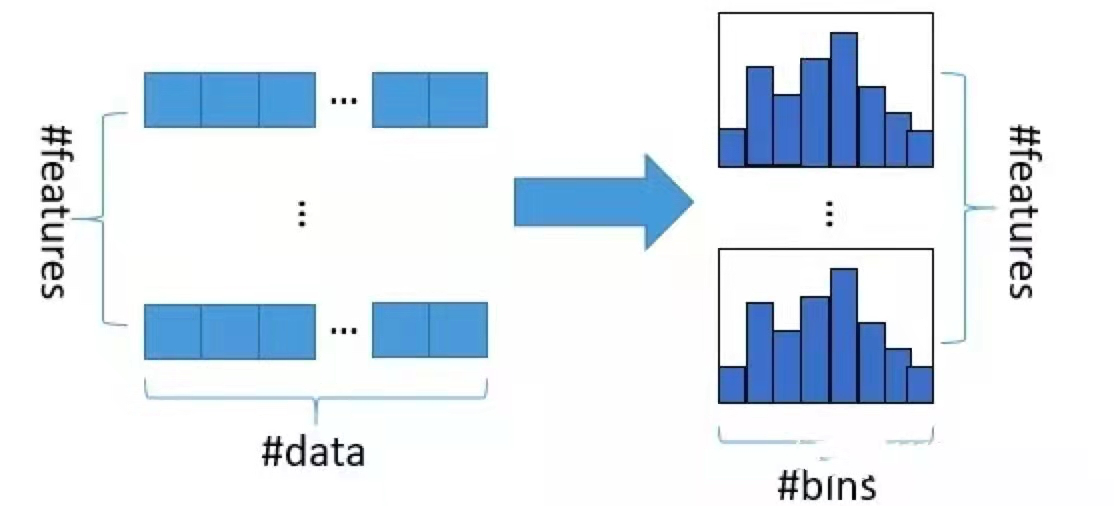• 記憶體佔用更小：直方圖演算法不僅不需要額外儲存預排序的結果，而且可以只儲存特徵離散化後的值，而這個值一般用位整型儲存就足夠了，記憶體消耗可以降低為原來的。也就是說XGBoost需要用位的浮點數去儲存特徵值，並用位的整形去儲存索引，而 LightGBM只需要用位去儲存直方圖，記憶體相當於減少為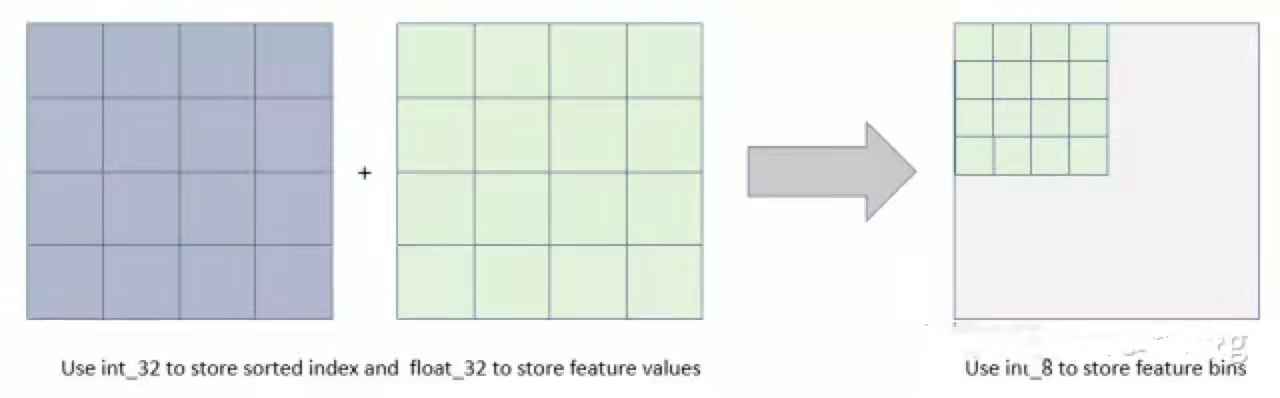• 計算代價更小：預排序演算法XGBoost每遍歷一個特徵值就需要計算一次分裂的增益，而直方圖演算法LightGBM只需要計算次（可以認為是常數），直接將時間複雜度從降低到，而我們知道LightGBM另一個優化是Histogram（直方圖）做差加速。一個葉子的直方圖可以由它的父親節點的直方圖與它兄弟的直方圖做差得到，在速度上可以提升一倍。通常構造直方圖時，需要遍歷該葉子上的所有資料，但直方圖做差僅需遍歷直方圖的k個桶。在實際構建樹的過程中，LightGBM還可以先計算直方圖小的葉子節點，然後利用直方圖做差來獲得直方圖大的葉子節點，這樣就可以用非常微小的代價得到它兄弟葉子的直方圖。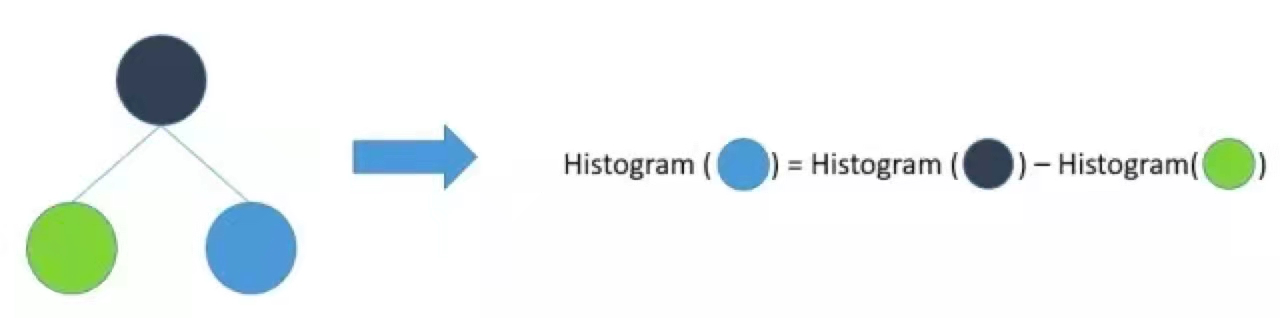XGBoost 採用 Level-wise 的增長策略，該策略遍歷一次資料可以同時分裂同一層的葉子，容易進行多執行緒優化，也好控制模型複雜度，不容易過擬合。但實際上Level-wise是一種低效的演算法，因為它不加區分的對待同一層的葉子，實際上很多葉子的分裂增益較低，沒必要進行搜尋和分裂，因此帶來了很多沒必要的計算開銷。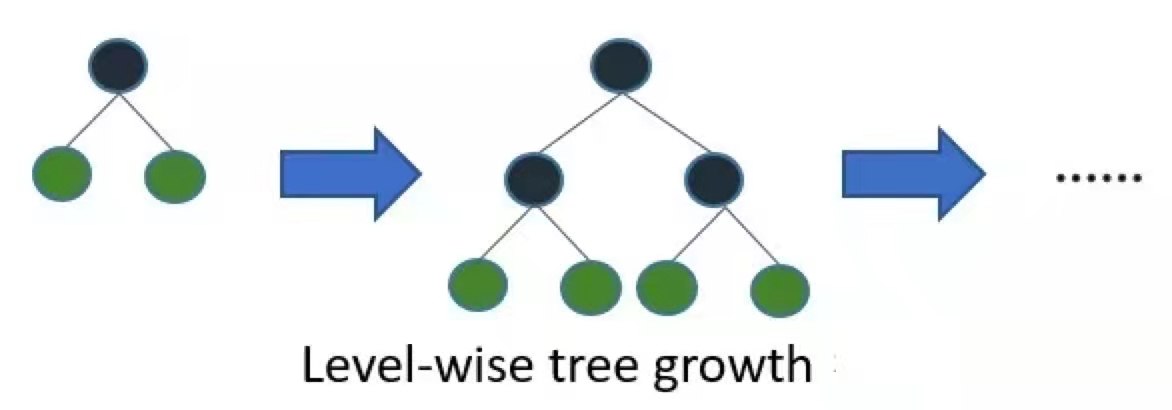LightGBM採用Leaf-wise的增長策略，該策略每次從當前所有葉子中，找到分裂增益最大的一個葉子，然後分裂，如此迴圈。因此同Level-wise相比，Leaf-wise的優點是：在分裂次數相同的情況下，Leaf-wise可以降低更多的誤差，得到更好的精度；Leaf-wise的缺點是：可能會長出比較深的決策樹，產生過擬合。因此LightGBM會在Leaf-wise之上增加了一個最大深度的限制，在保證高效率的同時防止過擬合。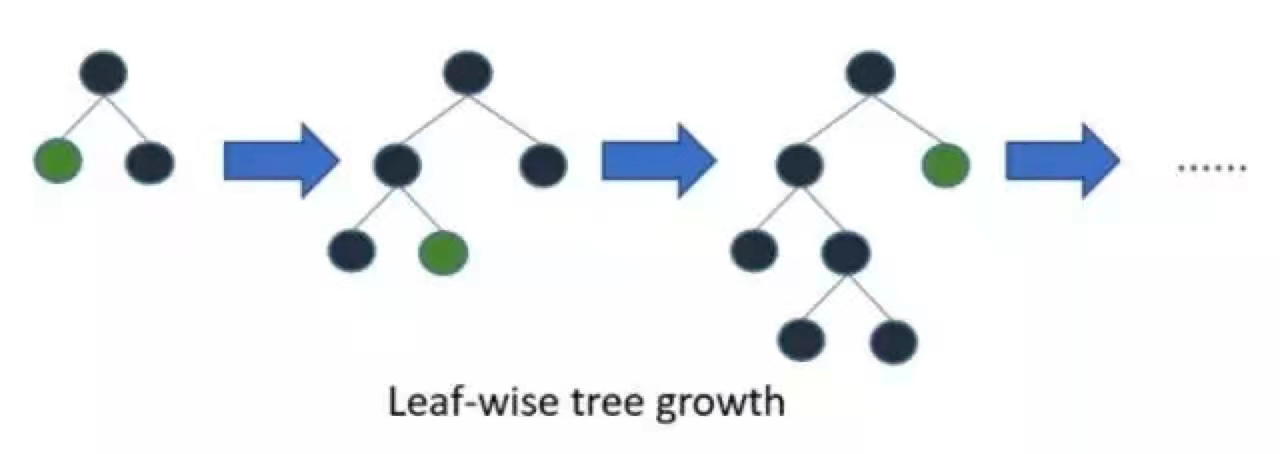「其他文章」